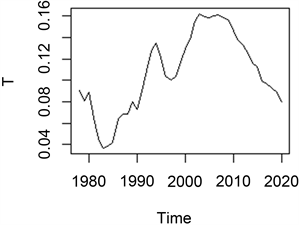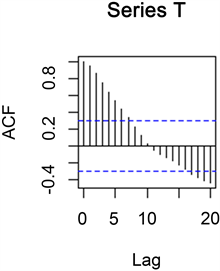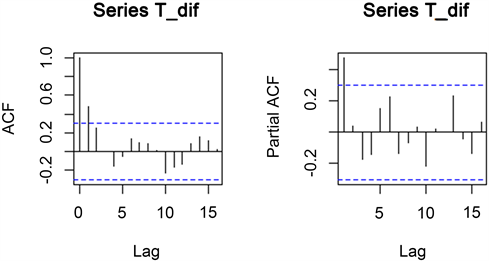#### 期刊菜单

Analysis and Forecast of Urban-Rural Income Gap Based on Theil Index
DOI: 10.12677/SA.2022.112046, PDF, HTML, XML, 下载: 67  浏览: 101

Abstract: In this paper, Theil index is selected to measure the urban-rural income gap in China. Based on the data from 1978 to 2018, the time series of Theil index is calculated and the ARIMA(1,1,0) model is established to predict Theil index from 2019 to 2025, and the predicted value from 2019 to 2021 is compared with the actual value. The results show that the error is small and the prediction effect is good. This paper draws the following conclusions: The urban-rural income gap in China is expected to narrow in the next few years, but the trend is relatively slow, which indicates that China is effective in the governance of urban-rural income balance and the implementation of relevant policies.

1. 研究背景

2. 研究现状

$T=\sqrt{2\left[{\left({x}_{1}-0.5\right)}^{2}+{\left({x}_{2}-0.5\right)}^{2}\right]}$

3. ARIMA模型

3.1. ARIMA模型

ARIMA模型具有如下结构 ，简记为ARIMA(p,d,q)模型：

$\left\{\begin{array}{l}\Phi \left(B\right){\nabla }^{d}{x}_{t}=\Theta \left(B\right){\epsilon }_{t}\hfill \\ E\left({\epsilon }_{t}\right)=0,Var\left({\epsilon }_{t}\right)={\sigma }_{\epsilon }^{2},E\left({\epsilon }_{t}{\epsilon }_{s}\right)=0,s\ne t\hfill \\ E\left({x}_{t}{\epsilon }_{t}\right)=0,\forall s (1)

3.2. 参数估计与模型检验

${\epsilon }_{t}=\frac{\Phi \left(B\right)}{\Theta \left(B\right)}{x}_{t}={x}_{t}-\underset{i=1}{\overset{t}{\sum }}{\pi }_{i}{x}_{t-i}$ (2)

$Q\left(\stackrel{˜}{\beta }\right)=\underset{i=1}{\overset{n}{\sum }}{\epsilon }_{t}{}^{2}=\underset{i=1}{\overset{n}{\sum }}{\left[{x}_{t}-\underset{i=1}{\overset{t}{\sum }}{\pi }_{i}{x}_{t-i}\right]}^{2}$ (3)

$LB=n\left(n+2\right)\underset{k=1}{\overset{m}{\sum }}\frac{{\stackrel{^}{\rho }}^{2}{}_{k}}{n-k}~{\chi }^{2}\left(m\right),\forall m>0$ (4)

$t=\frac{{\stackrel{^}{\varphi }}_{j}}{{\stackrel{^}{\sigma }}_{j}}~t\left(n-m\right)$ (5)

3.3. 建模步骤

1) 对原始序列进行平稳性检验，非平稳时间序列经过适当差分使其变成平稳序列；

2) 根据自相关系数和偏自相关系数确定模型阶数，初步识别模型的可能形式，然后根据AIC、BIC信息准则，从中选择一个最佳模型；

3) 参数估计与模型检验，模型检验指模型和参数的显著性检验，如果模型通过检验，则模型设定基本正确，否则，必须重新确定模型形式；

4) 用建立的ARIMA模型进行预测。

4. 数据来源

$T={\sum }_{j}\frac{{I}_{j}}{I}×\mathrm{ln}\left(\frac{{I}_{j}/I}{{N}_{j}/N}\right)$ (6)

5. 城乡收入差距模型的建立与预测Table 1. Theil index table from 1978 to 2021Table 2. Descriptive statistics of Theil index

5.1. 原始序列的平稳性检验Figure 1. Sequence T sequence diagramFigure 2. Sequence T autocorrelation diagram

5.2. 非平稳数据的处理与检验

5.3. 模型识别Figure 3. Sequence diagram of Theil index first order difference sequence T_difTable 3. Results of Box-Ljung test for first-order difference sequence of Theil indexTable 4. Basic principles of ARMA model recognitionFigure 4. Autocorrelator and partial autocorrelator of Theil index sequence after first order difference

5.4. 模型参数估计与检验

$\nabla {x}_{t}=0.4723\nabla {x}_{t-1}+{\epsilon }_{t}$ (7)Table 6. White noise test results of residual sequence of fitting model

5.5. 模型的预测Figure 5. Forecast figureTable 8. Comparison of predicted value with actual value

6. 结论及建议

  袁航. 基于ARMA模型对我国城乡居民收入差距的分析与预测[J]. 生产力研究, 2014(6): 121-124+155.  李焕焕, 武新乾. 中国城乡人均收入差异速率变化的统计建模[J]. 农村经济与科技, 2017, 28(17): 126-128.  蔡武, 吴加广. 城乡收入差距的ARMA模型预测与分析[J]. 统计与咨询, 2018(2): 20-23.  张延群, 万海远. 我国城乡居民收入差距的决定因素和趋势预测[J]. 数量经济技术经济研究, 2019, 36(3): 59-75.  王雨晨. 我国城乡居民收入差距动态研究——基于ARIMA模型[J]. 现代农业研究, 2020, 26(6): 28-29.  廖龙静, 王玉梅. 安徽省城乡收入差距组合预测研究[J]. 牡丹江师范学院学报(自然科学版), 2019(2): 7-10+30.  王燕. 应用时间序列分析[M]. 北京: 中国人民大学出版社, 2020.